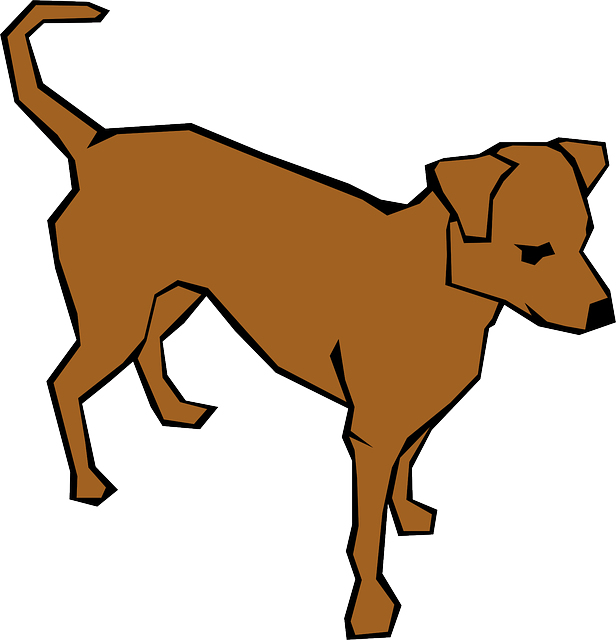10 minutes maximum! Can you do it in 5?These questions are all about the time it takes a vehicle to brake in an emergency. In this example a dog has run into the road, and the driver needs to stop suddenly. 1. Which of these graphs best shows the velocity of the bus from the moment the driver first sees the dog?ABCD 2. The driver will take some time to react. A reasonable estimate of human reaction time is: A. 0.03 s B. 0.3 s C. 1 s D. 2 s ABCD 3. Which of these correctly states the formula for working out the distance travelled before stopping? A. Total stopping distance = thinking distance × braking distance B. Total stopping distance = thinking distance - braking distance C. Total stopping distance = thinking distance + braking distance D. Total stopping distance = thinking distance ÷ braking distance ABCD 4-8: Here is a graph showing the buses' velocity when doing an emergency stop:Use this graph for the following questions: 4. How do you find the stopping distance of this vehicle? A. Use the formula : Maximum speed x time to stop. B. Calculate the area under the line. C. Read the total time taken to stop from the graph. D. Find the gradient of the line. ABCD 5. What was the 'thinking distance' of this vehicle? (The distance travelled whilst the driver reacts). A. 12 m B. 24 m C. 36 m D. 48 m ABCD 6. What was the 'braking distance' of this vehicle? (The distance travelled whilst the driver is braking). A. 12 m B. 24 m C. 36 m D. 48 m ABCD 7. What was the 'total stopping distance' of this vehicle? A. 12 m B. 24 m C. 36 m D. 48 m ABCD 8. What was the deceleration of the vehicle in the last 2 seconds? A. 12 m/s2 B. 24 m/s2 C. 3 m/s2 D. 6 m/s2 ABCD 9. This driver took some time to react. This may be due to A. the condition of the brakes. B. the driver having drunk alcohol. C. the high speed of the vehicle. D. the high mass of the vehicle. ABCD 10. Which of these would NOT affect the braking distance of a truck?A. Water on the road. B. The speed of the truck. C. The driver taking drugs such as cough mixtures. D. The condition of the tyres. ABCD